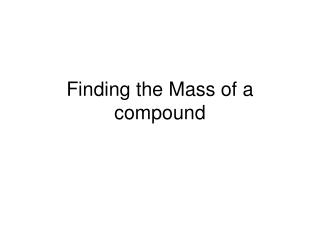# Finding the Mass of a compound - PowerPoint PPT PresentationDownload PresentationFinding the Mass of a compound

Finding the Mass of a compound
Download Presentation## Finding the Mass of a compound

- - - - - - - - - - - - - - - - - - - - - - - - - - - E N D - - - - - - - - - - - - - - - - - - - - - - - - - - -
##### Presentation Transcript

1. Finding the Mass of a compound

2. Formula Mass • Add up all the atomic masses in the compound’s formula Also called molecular mass if the compound is covalently bonded (forms molecules) (Remember, ionic compounds have no molecules)

3. Formula mass of NaCl: Look up atomic mass of Na: 23 amu Look up atomic mass of Cl: 35.3 amu

4. Formula mass of NaCl: Add the atomic masses together: 23 amu + 35.5 amu = 58.5 amu

5. Formula mass of K2CO3: This time you have: two K one C three O

6. Formula mass of K2CO3: Multiply the atomic mass by the number of atoms of that element in the formula K 39.1 x 2 = 78.2 amu C 12.0 x 1 = 12.0 amu O 16.0 x 3 = 48.0 amu

7. Formula mass of K2CO3: Now add the total mass for each element in the compound K 39.1 x 2 = 78.2 amu C 12.0 x 1 = 12.0 amu O 16.0 x 3 = 48.0 amu + ________ Formula Mass : 138.2 amu

8. Now you try it for: Mg (NO3)2

9. Now you try it for: Mg (NO3)2 Element# of atoms Mg 1 N 2 O 6

10. Now you try it for: Mg (NO3)2 Element# of atoms Atomic mass Mg 1 24.3 amu N 2 14.0 amu O 6 16.0 amu

11. Now you try it for: Mg (NO3)2 Mg 1 x 24.3 = 24.3 amu N 2 x 14.0 = 28.0 amu O 6 x 16.0 = 96.0 amu

12. Mg (NO3)2 Mg 1 x 24.3 = 24.3 amu N 2 x 14.0 = 28.0 amu O 6 x 16.0 = 96.0 amu +_______ 148.3 amu

13. Gram Formula Mass (GFM) is the formula mass expressed in grams instead of in amu This is a much more useful and practical unit Follow the same steps as formula mass, except your answer will have units of grams, not amu

14. Formulas of Hydrates A HYDRATE is a an ionic compound that has water molecules attached to its crystal structure in the solid phase. These water molecules are weakly attached to the crystal, and are easily removed if the crystals are heated. Once the water is removed, the compound is called ANHYDROUS (without water).

15. Formulas of Hydrates Na2CO3• 10 H2O This is a sodium carbonate crystal with ten water molecules attached to every one sodium carbonate The • is the symbol that “attaches” the ten water molecules to the sodium carbonate. Think of it as a “+”

16. Finding GFM of Hydrates Na2CO3• 10 H2O Type # of atomsatomic mass Na 2 23 C 1 12 O 3 16 H2O 10 2 + 16 = 18

17. Finding GFM of Hydrates Na2CO3• 10 H2O Na 2 x 23 = 46 C 1 x 12 = 12 O 3 x 16 = 48 H2O 10 x 18 = + 180 286 g

18. You try it! Find the GFM of the hydrated crystal CaSO4• 2H2O

19. You try it! CaSO4• 2H2O Ca 40 x 1 = 40 S 32 x 1 = 32 O 16 x 4 = 64 H2O 18 x 2 = + 36 172 g